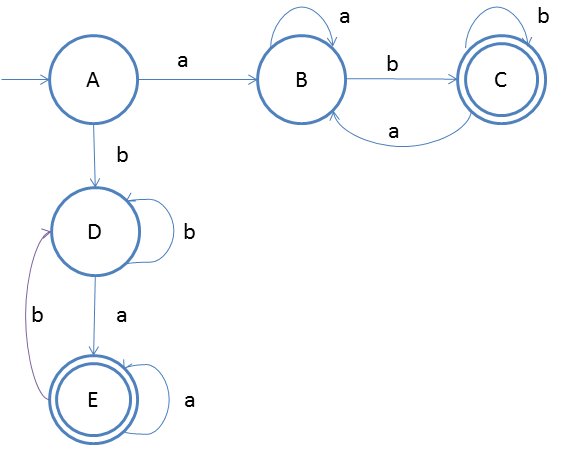# DFA String Examples

We will now discuss about string patterns such as, starting with some symbol, ending with some symbol, etc.

Design a DFA in which start and end symbol must be different
Given: Input alphabet, Σ={a, b}
Language L = {ab, ab, abab, aabb, aaabbb,...}

Clearly the language is infinite because there is infinite number of strings.The idea behind this approach is very simple, follow the steps below and you will understand.

1. We know that if the first input is 'a' then the last input cannot be 'a', so we start two flows form start state, one for staring with 'a' and another for starting with 'b'
2. This is also clear that there will be two final states
3. In the first flow starting with 'a' we have already discussed the process in the previous examples. We will accept the input 'a' on state B for multiple times and then on b we will go to final state.
4. On final state we will direct the input 'a' to state B so that the last symbol should not be 'a'
5. The same mechanism will be for another flow staring from start state A on input 'a'.

### Testing 1

1. Lets take one input string abab(this will describe for strings which starts with a and ends with different symbol b)
2. Scan string from left to right
3. First input is a, so from state A we will go to state B
4. Second input is b, so from state B we will go to state C
5. Third input is a, so from state C we will go to state B
6. Fourth input is b, so from state B we will go to state C (final state)
After end of the string we are at final state so string is accepted.

### Testing 2

1. Lets take another input string bbabaa(this will describe for strings which starts with b and ends with different symbol a)
2. Scan string from left to right
3. First input is b, so from state A we will go to state D
4. Second input is b, so from state D we will go to state D itself
5. Third input is a, so from state D we will go to state E
6. Fourth input is b, so from state E we will go to state D
7. Fifth input is a, so from state D we will go to state E (final state)
8. Sixth input is a, so from state E we will go to state E itself(final state)
After end of the string we are at final state so string is accepted.
You may also try some examples of strings to run over this DFA, and you will understand more